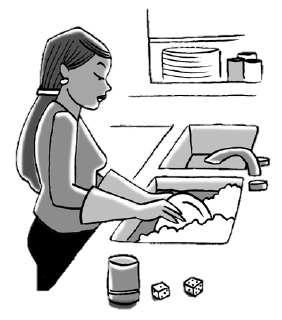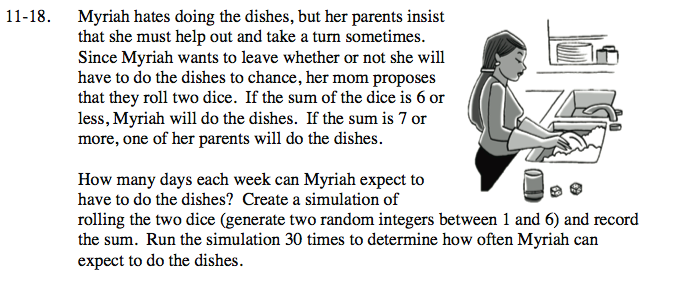### Home > CCA2 > Chapter Ch11 > Lesson 11.1.2 > Problem11-18

11-18.
1.Myriah hates doing the dishes, but her parents insist that she must help out and take a turn sometimes. Since Myriah wants to leave whether or not she will have to do the dishes to chance, her mom proposes that they roll two dice. If the sum of the dice is 6 or less, Myriah will do the dishes. If the sum is 7 or more, one of her parents will do the dishes.

2. How many days each week can Myriah expect to have to do the dishes? Create a simulation of rolling the two dice (generate two random integers between 1 and 6) and record the sum. Run the simulation 30 times to determine how often Myriah can expect to do the dishes. Homework Help ✎Use a random number generator to generate 60 random integers from 1 to 6.

Record the sum of each pair of numbers (60 numbers is 30 pairs).

How many pairs of numbers have a sum of 7 or more?